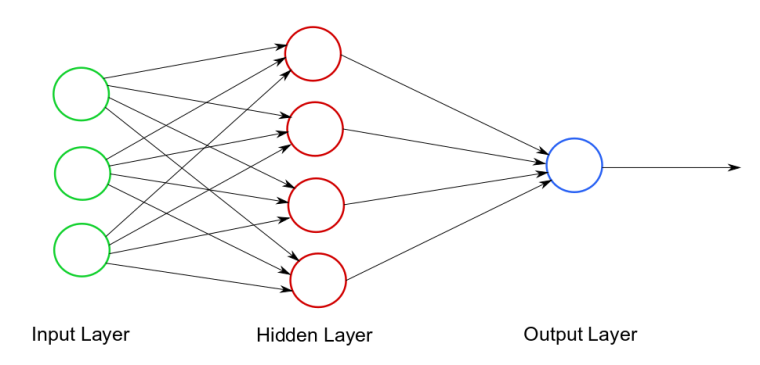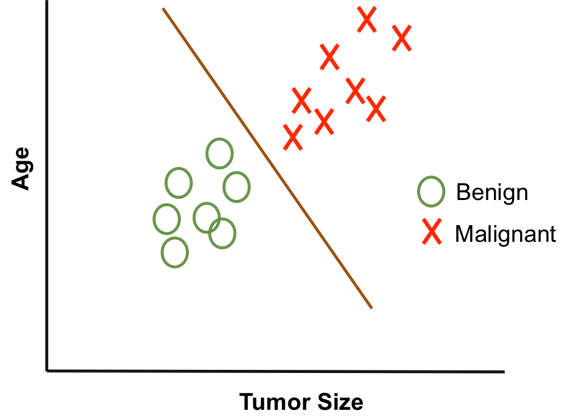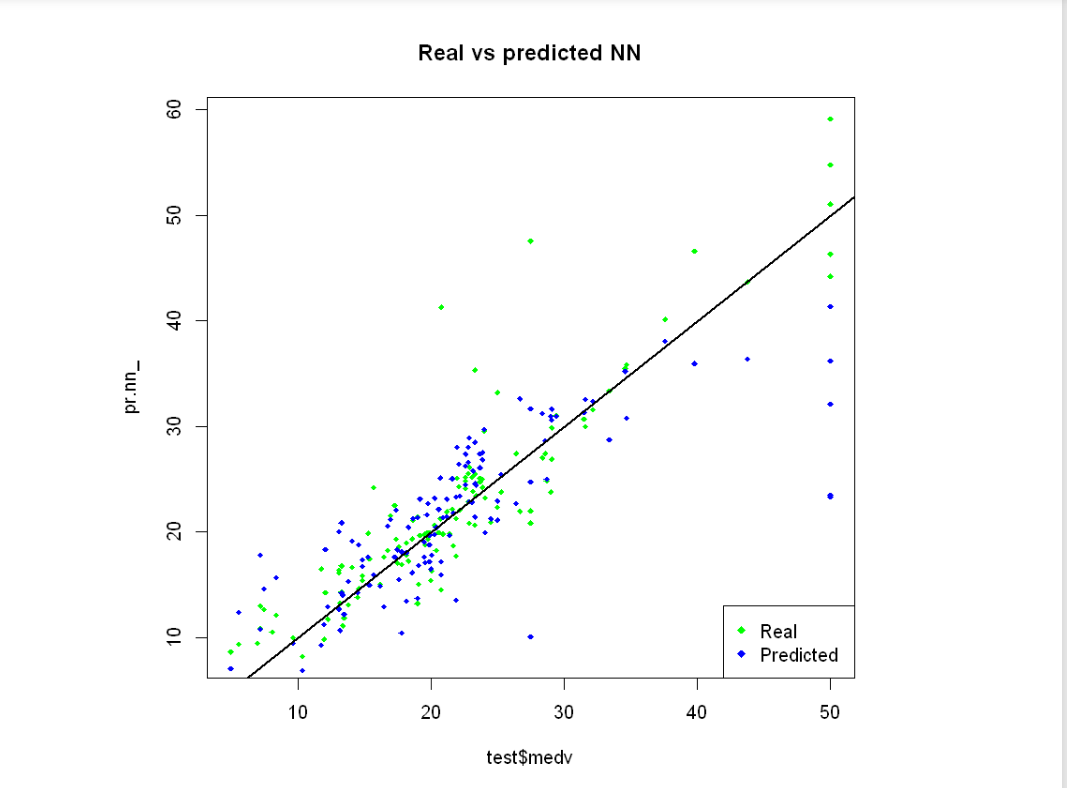# How Neural Networks are used for Classification in R Programming

Neural Networks is a well known word in machine learning and data science. Neural networks are used almost in every machine learning application because of its reliability and mathematical power. In this article let’s deal with applications of neural networks in classification problems by using R programming. First briefly look at neural network and classification algorithms and then combine both the concepts.

#### Neural Network

The neural network is a general circuit of neurons that can work on any number of inputs and are usually suitable for dealing with nonlinear datasets. Neural networks are more flexible and can be used with both regression and classification problems. So imagine a situation in which we are supposed to train a model and check if we are on the right track or not, hence we repeatedly forward propagate and backpropagate to get the highest accuracy (generally using epochs), this whole procedure is nothing but working on a neural network! Following is a general visualization of a neural network.#### Classification

Classification is a powerful tool for working with discrete data. Most of the True/False or Yes/No type machine learning problems are solved using classification. Predicting whether an email is a spam or not spam is a famous example of binary classification. Other examples include classifying breast cancer as malignant or benign, classifying handwritten characters, etc. Following is a general visualization of a classification.Now as we have become familiar with neural network and classification algorithms, let’s deal with the application of neural networks in classification. Neural Network classification is widely used in image processing, handwritten digit classification, signature recognition, data analysis, data comparison, and many more. The hidden layers of the neural network perform epochs with each other and with the input layer for increasing accuracy and minimizing a loss function.

#### Implementation in R

Let us construct a simple neural network in R and visualize the real and predicted values of the neural network. For this example, let’s take R’s inbuilt Boston data set.

Example:

 `# Generating random number ` `# Using set.seed() ` `set.seed``(500) ` ` `  `# Import required library ` `library``(MASS) ` ` `  `# Import data set ` `data <- Boston ` `head``(data) `

```    crim  zn indus   chas nox  rm    age  dis   rad tax  ptratio black lstat medv
1 0.00632 18  2.31    0 0.538 6.575 65.2 4.0900   1 296    15.3 396.90  4.98 24.0
2 0.02731  0  7.07    0 0.469 6.421 78.9 4.9671   2 242    17.8 396.90  9.14 21.6
3 0.02729  0  7.07    0 0.469 7.185 61.1 4.9671   2 242    17.8 392.83  4.03 34.7
4 0.03237  0  2.18    0 0.458 6.998 45.8 6.0622   3 222    18.7 394.63  2.94 33.4
5 0.06905  0  2.18    0 0.458 7.147 54.2 6.0622   3 222    18.7 396.90  5.33 36.2
6 0.02985  0  2.18    0 0.458 6.430 58.7 6.0622   3 222    18.7 394.12  5.21 28.7
```

Now let’s fit the dataset to test and train set, as follows:

 `# Split the dataset to ` `# Test and train set ` `index <- ``sample``(1:``nrow``(data),  ` `         ``round``(0.75 * ``nrow``(data))) ` `train <- data[index, ] ` `test <- data[-index, ] ` ` `  `# Fit the model ` `lm.fit <- ``glm``(medv~., data = train) ` `summary``(lm.fit) ` `pr.lm <- ``predict``(lm.fit, test) ` `MSE.lm <- ``sum``((pr.lm - test\$medv)^2) / ``nrow``(test) `

After fitting and dividing our dataset, now prepare the neural network to fit in, this can be done as follows:

 `# Fit the neural network ` `maxs <- ``apply``(data, 2, max)  ` `mins <- ``apply``(data, 2, min) ` ` `  `scaled <- ``as.data.frame``(``scale``(data,  ` `                              ``center = mins, ` `                              ``scale = maxs - mins)) ` ` `  `train_ <- scaled[index, ] ` `test_ <- scaled[-index, ] `

Now work on parameters like hidden layers of neural network using `neuralnet` library, as follows.

 `# Import neuralnet library ` `library``(neuralnet) ` ` `  `# Work on parameters  ` `# of hidden layers of NN ` `n <- ``names``(train_) ` `f <- ``as.formula``(``paste``(``"medv ~"``,  ` `                ``paste``(n[! n % ``in` `% ``"medv"``],  ` `                ``collapse = ``" + "``))) ` `nn <- ``neuralnet``(f,  ` `                ``data = train_,  ` `                ``hidden = ``c``(5, 3), ` `                ``linear.output = T) `

After this, compile our function for Predicting medv using the neural network as follows:

 `# Predicting the medv ` `pr.nn <- ``compute``(nn, test_[, 1:13]) ` ` `  `pr.nn_ <- pr.nn\$net.result * (``max``(data\$medv) ` `                           ``- ``min``(data\$medv)) ` `                           ``+ ``min``(data\$medv) ` `test.r <- (test_\$medv) * (``max``(data\$medv) ` `                       ``- ``min``(data\$medv)) ` `                       ``+ ``min``(data\$medv) ` ` `  `MSE.nn <- ``sum``((test.r - pr.nn_)^2) / ``nrow``(test_) `

Now let us plot the final graph to visualize the neural network for real values and predicted values.

 `# Plotting the final graph ` `plot``(test\$medv, pr.nn_,  ` `                ``col = ``'green'``,  ` `                ``main = ``'Real vs predicted NN'``, ` `                ``pch = 18, cex = 0.7) ` `points``(test\$medv, pr.lm,  ` `                  ``col = ``'blue'``,  ` `                  ``pch = 18, cex = 0.7) ` `abline``(0, 1, lwd = 2) ` `legend``(``'bottomright'``,  ` `        ``legend = ``c``(``'Real'``, ``'Predicted'``), ` `        ``pch = 18,  ` `        ``col = ``c``(``'green'``, ``'blue'``)) `

Output:My Personal Notes arrow_drop_upEnthusiast Technical Content Writer

If you like GeeksforGeeks and would like to contribute, you can also write an article using contribute.geeksforgeeks.org or mail your article to contribute@geeksforgeeks.org. See your article appearing on the GeeksforGeeks main page and help other Geeks.

Please Improve this article if you find anything incorrect by clicking on the "Improve Article" button below.

Article Tags :

1

Please write to us at contribute@geeksforgeeks.org to report any issue with the above content.